# Aircraft Gas Turbine Engine Performance

Thermal efficiency is a prime factor in gas turbine performance. It is the ratio of net work produced by the engine to the chemical energy supplied in the form of fuel. The three most important factors affecting the thermal efficiency are turbine inlet temperature, compression ratio, and the component efficiencies of the compressor and turbine. Other factors that affect thermal efficiency are compressor inlet temperature and combustion efficiency. Figure 1 shows the effect that changing compression ratio (compressor pressure ratio) has on thermal efficiency when compressor inlet temperature and the component efficiencies of the compressor and turbine remain constant. The effects that compressor and turbine component efficiencies have on thermal efficiency when turbine and compressor inlet temperatures remain constant are shown in Figure 2. In actual operation, the turbine engine exhaust temperature varies directly with turbine inlet temperature at a constant compression ratio.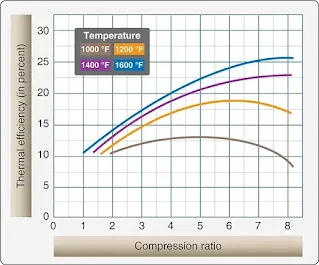Figure 1. The effect of compression ratio on thermal efficiency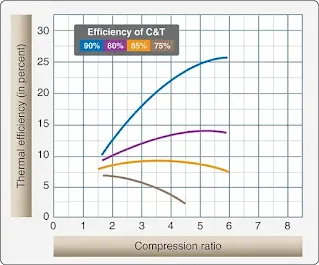Figure 2. Turbine and compressor efficiency vs. thermal efficiency

Rpm is a direct measure of compression ratio; therefore, at constant rpm, maximum thermal efficiency can be obtained by maintaining the highest possible exhaust temperature. Since engine life is greatly reduced at high turbine inlet temperatures, the operator should not exceed the exhaust temperatures specified for continuous operation.

Figure 3 illustrates the effect of turbine inlet temperature on turbine blade life. In the previous discussion, it was assumed that the state of the air at the inlet to the compressor remains constant. Since this is a practical application of a turbine engine, it becomes necessary to analyze the effect of varying inlet conditions on the thrust or power produced. The three principal variables that affect inlet conditions are the speed of the aircraft, the altitude of the aircraft, and the ambient temperature. To make the analysis simpler, the combination of these three variables can be represented by a single variable called stagnation density.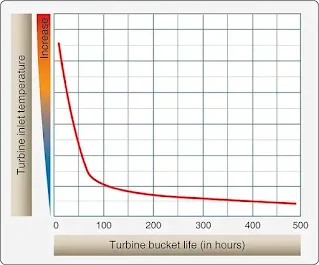Figure 3. Effect of turbine inlet temperature on turbine bucket life

The power produced by a turbine engine is proportional to the stagnation density at the inlet. The next three illustrations show how changing the density by varying altitude, airspeed, and outside air temperature affects the power level of the engine. Figure 4 shows that the thrust output improves rapidly with a reduction in outside air temperature (OAT) at constant altitude, rpm, and airspeed. This increase occurs partly because the energy required per pound of airflow to drive the compressor varies directly with the temperature, leaving more energy to develop thrust. In addition, the thrust output increases since the air at reduced temperature has an increased density. The increase in density causes the mass flow through the engine to increase. The altitude effect on thrust, as shown in Figure 5, can also be discussed as a density and temperature effect. In this case, an increase in altitude causes a decrease in pressure and temperature.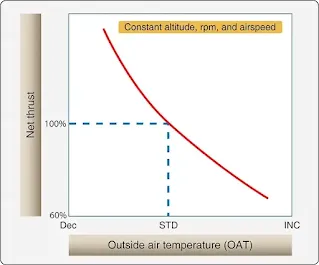Figure 4. Effect of OAT on thrust output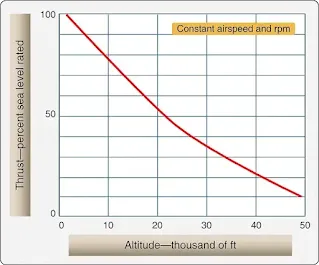Figure 5. Effect of altitude on thrust output

Since the temperature lapse rate is lower than the pressure lapse rate as altitude is increased, the density is decreased. Although the decreased temperature increases thrust, the effect of decreased density more than offsets the effect of the colder temperature. The net result of increased altitude is a reduction in the thrust output.

The effect of airspeed on the thrust of a gas-turbine engine is shown in Figure 6. To explain the airspeed effect, it is necessary to understand first the effect of airspeed on the factors that combine to produce net thrust: specific thrust and engine airflow. Specific thrust is the net thrust in pounds developed per pound of airflow per second. It is the remainder of specific gross thrust minus specific ram drag. As airspeed is increased, ram drag increases rapidly. The exhaust velocity remains relatively constant; thus, the effect of the increase in airspeed results in decreased specific thrust. [Figure 6] In the low-speed range, the specific thrust decreases faster than the airflow increases and causes a decrease in net thrust. As the airspeed increases into the higher range, the airflow increases faster than the specific thrust decreases and causes the net thrust to increase until sonic velocity is reached. The effect of the combination on net thrust is illustrated in Figure 7.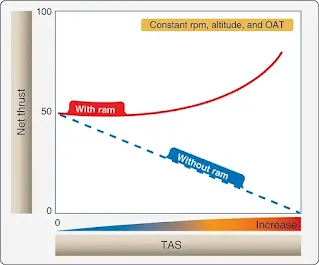Figure 6. Effect of airspeed on net thrust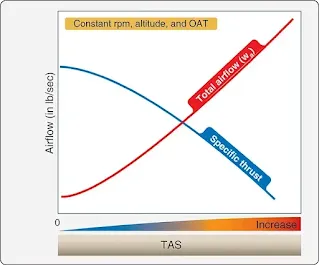Figure 7. Effect of airspeed on specific thrust and total engine airflow

## Ram Recovery

A rise in pressure above existing outside atmospheric pressure at the engine inlet, as a result of the forward velocity of an aircraft, is referred to as ram pressure. Since any ram effect causes an increase in compressor entrance pressure over atmospheric, the resulting pressure rise causes an increase in the mass airflow and gas velocity, both of which tend to increase thrust. Although ram effect increases engine thrust, the thrust being produced by the engine decreases for a given throttle setting as the aircraft gains airspeed. Therefore, two opposing trends occur when an aircraft’s speed is increased. What actually takes place is the net result of these two different effects.

An engine’s thrust output temporarily decreases as aircraft speed increases from static, but soon ceases to decrease. Moving toward higher speeds, thrust output begins to increase again due to the increased pressure of ram recovery.

RELATED POSTS